﻿ Flow in a T-shaped branch pipe - XSim

# Flow in a T-shaped branch pipe

Update: June 1, 2017
OpenFOAM 4.x

## Case directory

\$FOAM_TUTORIALS/incompressible/pimpleFoam/TJunction

## Summary

We calculate the flow in a T-shaped branch pipe from 0 sec to 1.5 sec.

We apply the total pressure condition to the region "inlet", set to 10 m2/s2 (= (kg/(m-s2))/(kg/m3) = Pa/density) at 0 sec and 40 m2/s2 at 1 sec. We also apply a static pressure of 10 m2/s2 and 0 m2/s2 to the outlet side regions "outlet1" and "outlet2" respectively. The channel wall will be set to the no-slip condition.Model geometry

The meshes are as follows, and the number of mesh is 3875.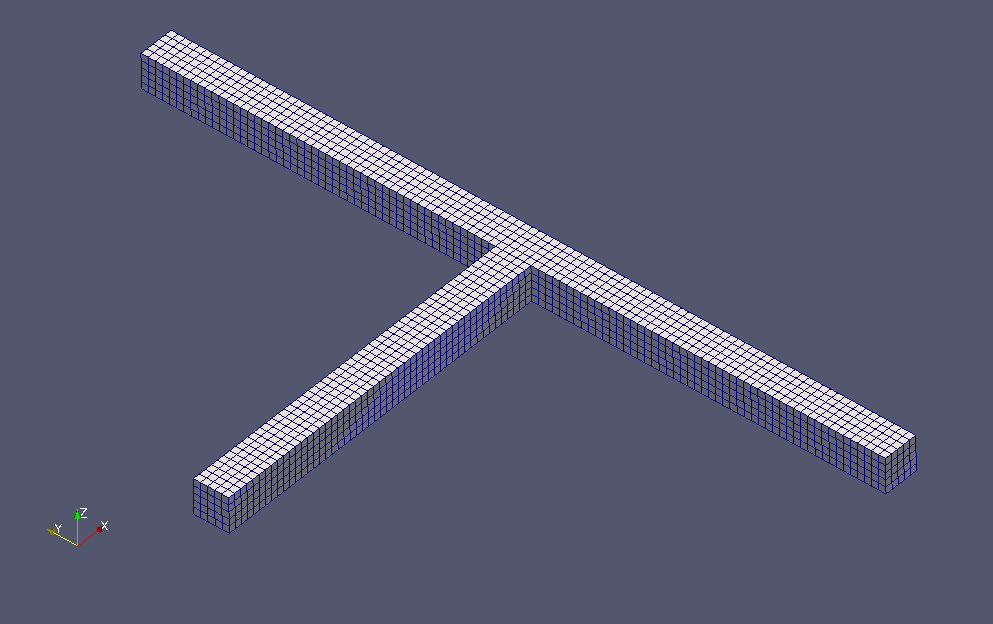Meshes

To visualize the calculated turbulence energy and turbulence dissipation rate, check "k" and "epsilon" in the "Properties" tab on ParaView.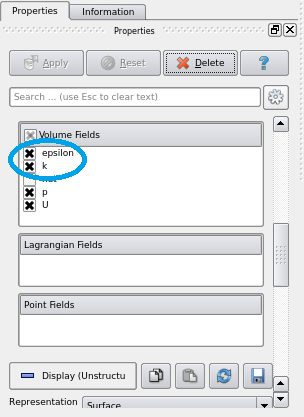Check "k" and "epsilon"

The calculation result is as follows.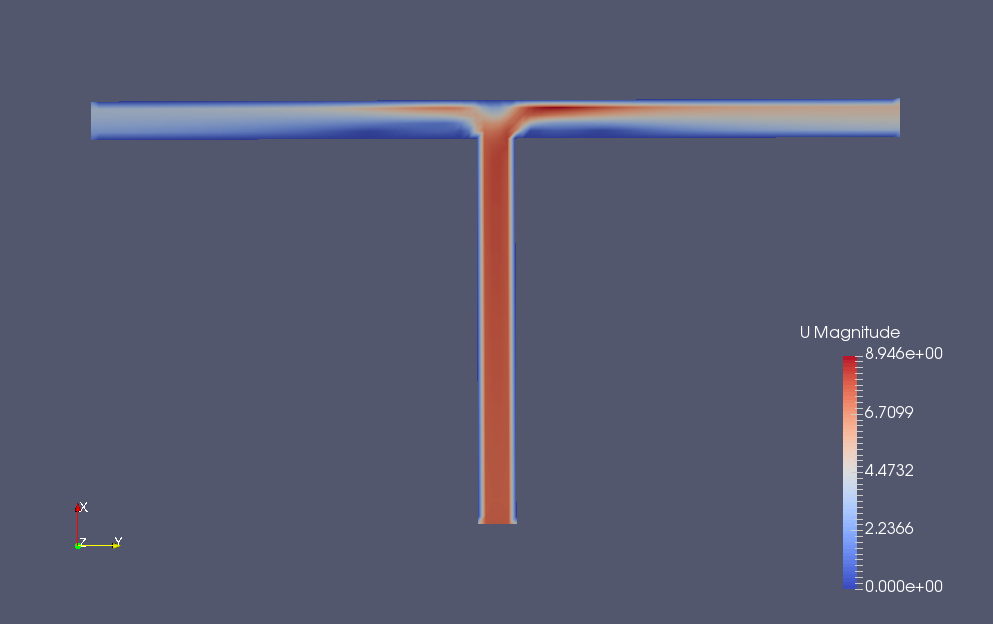Flow velocity at final time (U)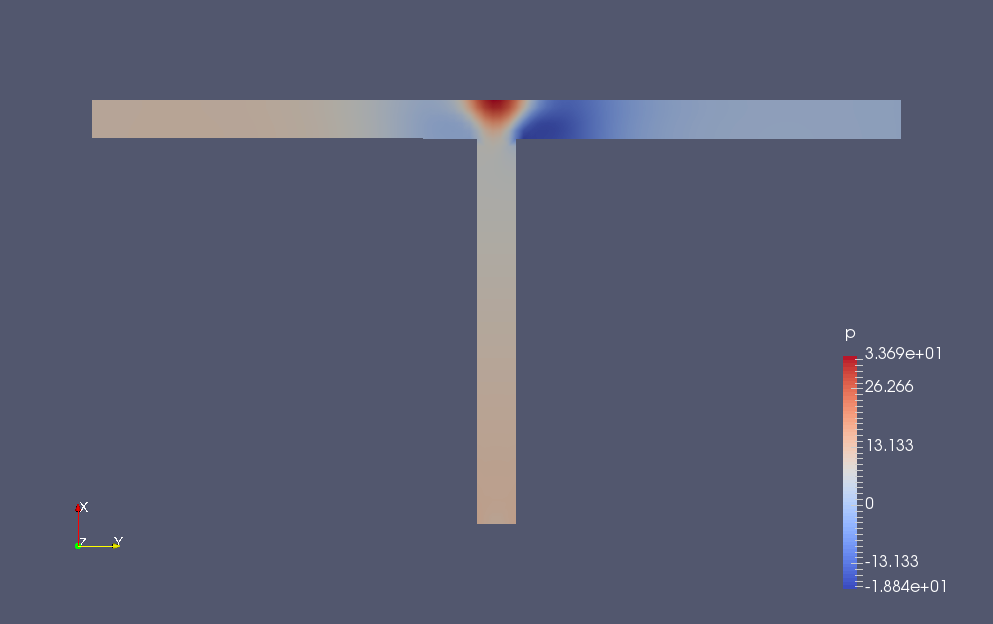Pressure at final time (p)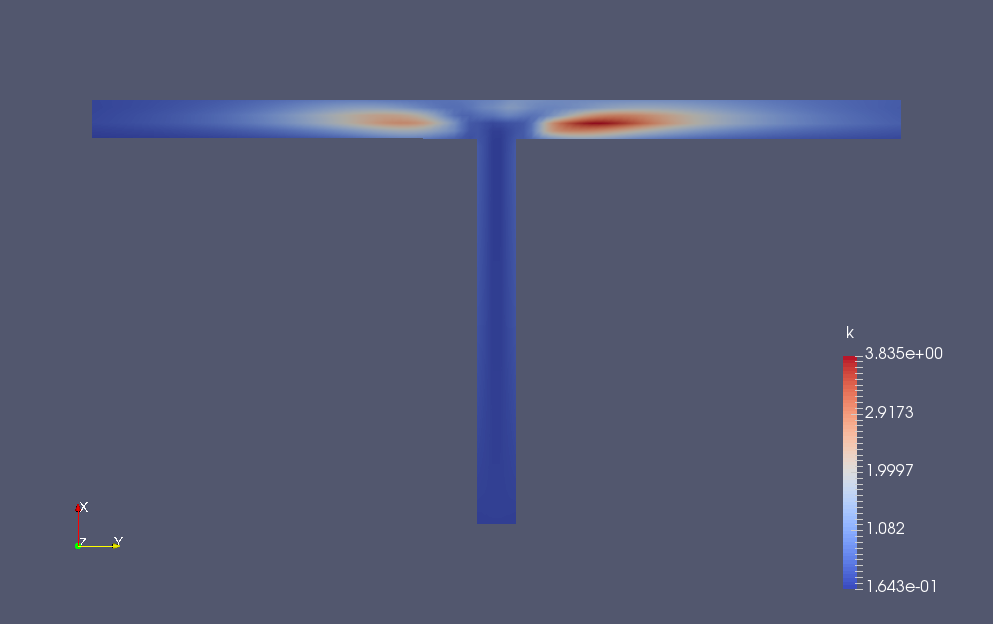Turbulence energy at final time (k)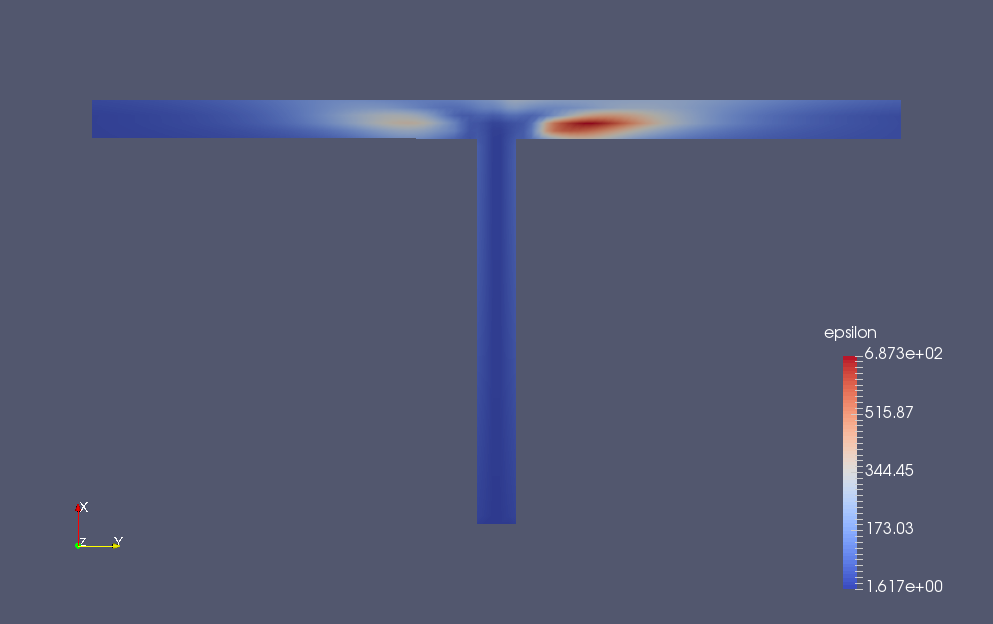Turbulence dissipation rate at final time (epsilon)

## Commands

cp -r \$FOAM_TUTORIALS/incompressible/pimpleFoam/TJunction TJunction
cd TJunction

blockMesh
pimpleFoam

paraFoam

## Calculation time

15.19 seconds *Single, Inter(R) Core(TM) i7-2600 CPU @ 3.40GHz 3.40GHz